# Areas of rectangles with the same perimeter Online Quiz

Following quiz provides Multiple Choice Questions (MCQs) related to Areas of rectangles with the same perimeter. You will have to read all the given answers and click over the correct answer. If you are not sure about the answer then you can check the answer using Show Answer button. You can use Next Quiz button to check new set of questions in the quiz.Q 1 - The rectangle below has the dimensions 8×2. Create a rectangle with the same perimeter, but a different area.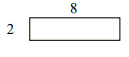### Explanation

Step 1:

Perimeter of given rectangle = 2(l + w) = 2(8 + 2)= 20; Area = l × w = 8 × 2 = 16 square units

Step 2:

One possible rectangle with same perimeter has dimensions 7×3: Its perimeter = 2(7 + 3) = 20

Its area that is different = 7×3 = 21 square units

Q 2 - The rectangle below has the dimensions 4×5. Create a rectangle with the same perimeter, but a different area.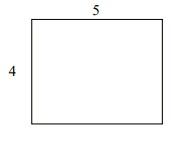### Explanation

Step 1:

Perimeter of given rectangle = 2(l + w) = 2(4 + 5) = 18; Area = l × w = 4 × 5 = 20 square units

Step 2:

One possible rectangle with same perimeter has dimensions 6×3. Its perimeter = 2(6 + 3) = 18

Its area that is different = 6×3 = 18 square units

Q 3 - The rectangle below has the dimensions 9×4. Create a rectangle with the same perimeter, but a different area.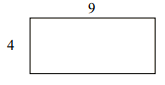### Explanation

Step 1:

Perimeter of given rectangle = 2(l + w) = 2(9 + 4) = 26; Area = l × w = 9 × 4 = 36 square units

Step 2:

One possible rectangle with same perimeter has dimensions 8×5; Its perimeter = 2(8 + 5) = 26

Its area that is different = 8×5 = 40 square units

Q 4 - The rectangle below has the dimensions 9×6. Create a rectangle with the same perimeter, but a different area.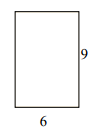### Explanation

Step 1:

Perimeter of given rectangle = 2(l + w) = 2(9 + 6) = 30; Area = l × w = 9 × 6 = 54 square units

Step 2:

One possible rectangle with same perimeter has dimensions 8×7; Its perimeter = 2(7 + 8) = 30

Its area that is different = 7×8 = 56 square units

Q 5 - The rectangle below has the dimensions 7×2. Create a rectangle with the same perimeter, but a different area.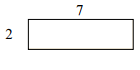### Explanation

Step 1:

Perimeter of given rectangle = 2(l + w) = 2(7 + 2) = 18; Area = l × w = 7 × 2 = 14 square units

Step 2:

One possible rectangle with same perimeter has dimensions 6×3; Its perimeter = 2(6 + 3) = 18

Its area that is different = 6×3 = 18 square units

Q 6 - The rectangle below has the dimensions 8×5. Create a rectangle with the same perimeter, but a different area.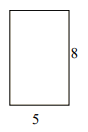### Explanation

Step 1:

Perimeter of given rectangle = 2(l + w) = 2(8 + 5) = 26; Area = l × w = 8 × 5 = 40 square units

Step 2:

One possible rectangle with same perimeter has dimensions 7×6; Its perimeter = 2(7 + 6) = 26

Its area that is different = 7×6 = 42 square units

Q 7 - The rectangle below has the dimensions 2×7. Create a rectangle with the same perimeter, but a different area.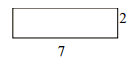### Explanation

Step 1:

Perimeter of given rectangle = 2(l + w) = 2(7 + 2) = 18; Area = l × w = 7 × 2 = 14 square units

Step 2:

One possible rectangle with same perimeter has dimensions 6×3; Its perimeter = 2(6 + 3) = 18

Its area that is different = 6×3 = 18 square units

Q 8 - The rectangle below has the dimensions 6×2. Create a rectangle with the same perimeter, but a different area.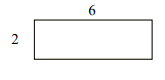### Explanation

Step 1:

Perimeter of given rectangle = 2(l + w) = 2(6 + 2) = 16; Area = l × w = 6 × 2 = 12 square units

Step 2:

One possible rectangle with same perimeter has dimensions 5×3; Its perimeter = 2(5 + 3) = 16

Its area that is different = 5×3 = 15 square units

Q 9 - The rectangle below has the dimensions 8×4. Create a rectangle with the same perimeter, but a different area.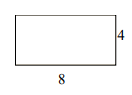### Explanation

Step 1:

Perimeter of given rectangle = 2(l + w) = 2(8 + 4) = 24; Area = l × w = 8 × 4 = 32 square units

Step 2:

One possible rectangle with same perimeter has dimensions 7×5; Its perimeter = 2(7 + 5) = 24

Its area that is different = 7×5 = 35 square units

Q 10 - The rectangle below has the dimensions 6×3. Create a rectangle with the same perimeter, but a different area.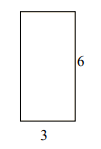### Explanation

Step 1:

Perimeter of given rectangle = 2(l + w) = 2(6 + 3) = 18; Area = l × w = 6 × 3 = 18 square units

Step 2:

One possible rectangle with same perimeter has dimensions 5×4; Its perimeter = 2(5 + 4) = 18

Its area that is different = 5×4 = 20 square units

areas_of_rectangles_with_same_perimeter.htm